# What is Similarity?

What is Similarity?

Maria: Dad, my teacher was saying that the Sun and Moon are not equal in size.'Maria: Then, why do they appear equal in size?

Dad: Due to their shape and distance. This feature is also called the property of similarity.

Would you like to know more?

In this mini-lesson, you will be introduced to the properties of similarity and its role in geometry.

## Lesson Plan

 1 What is Similarity? 2 Important notes on Similarity 3 Solved Examples of Similarity 4 Challenging Questions Similarity 5 Interactive Questions on Similarity

## What Do You Mean By Similarity?

When two or more objects or figures appear the same or equal due to their shape, this property is known as a similarity.

When we magnify or demagnify similar figures, they always superimpose each other.

For example, two circles (of any radii) will always superimpose each other because they are similar: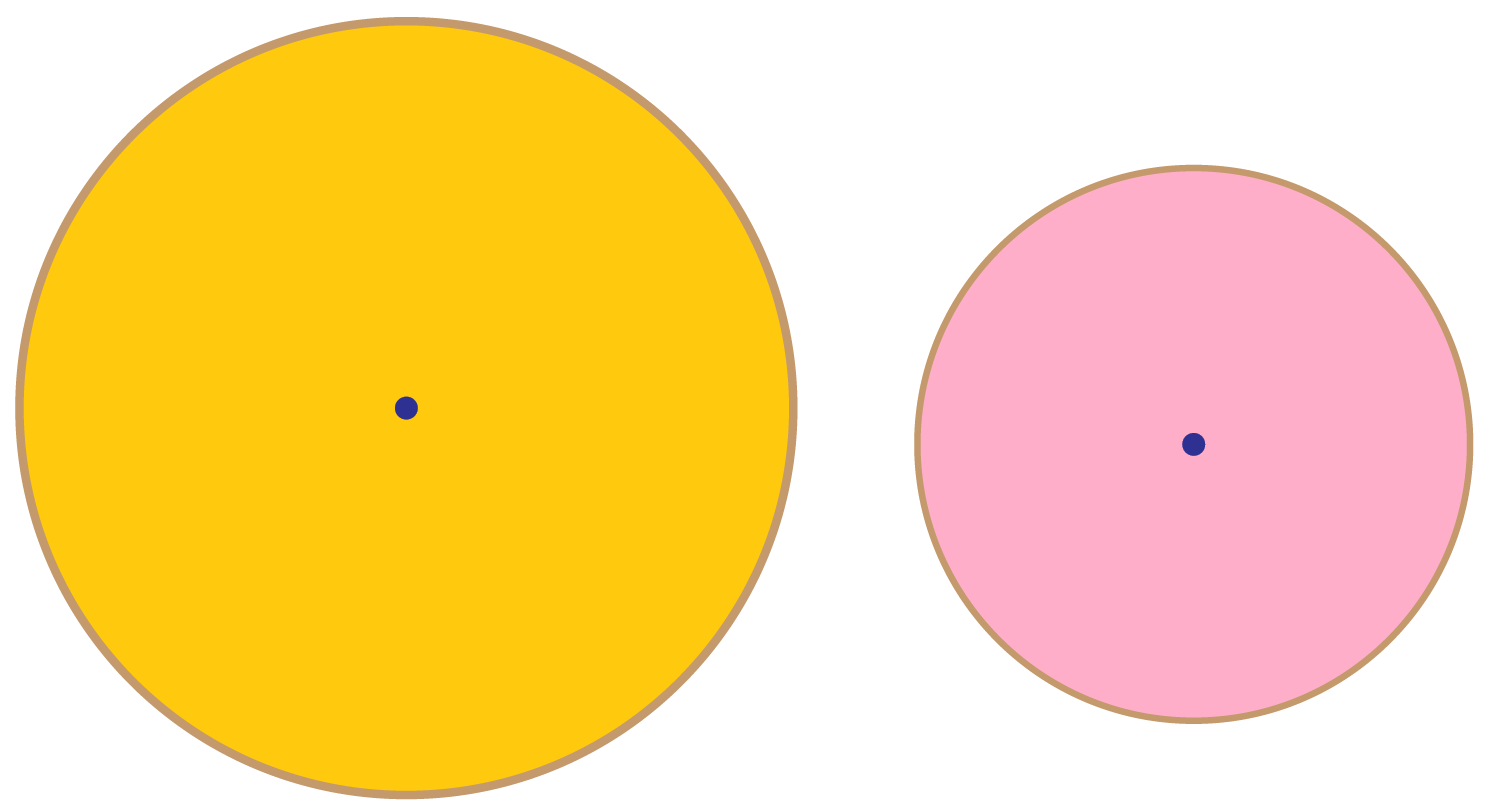1. In the same manner, two squares (of any side lengths) will always be similar: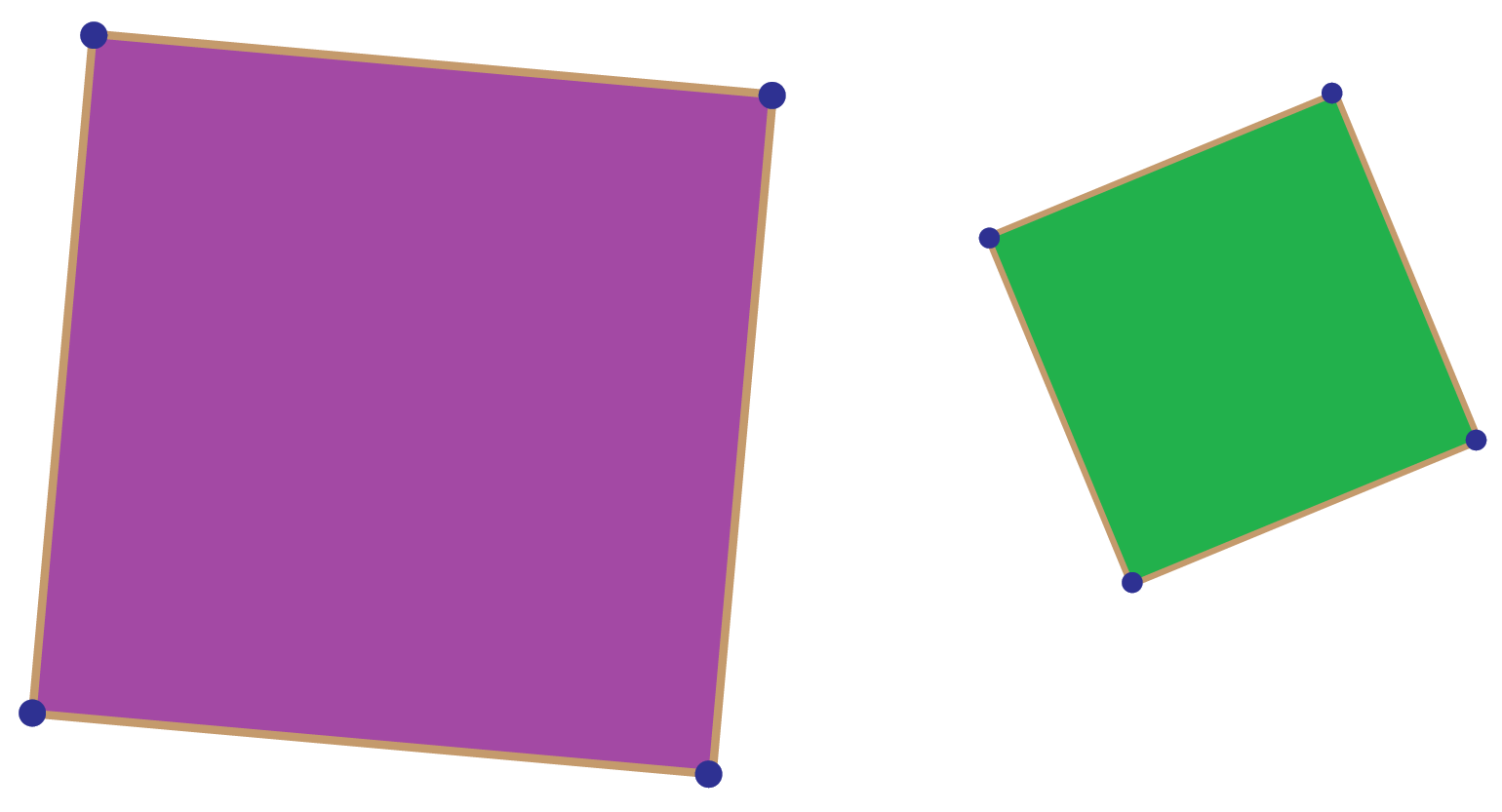## What Are The Rules Of Similarity?

If two linear figures are similar it means that:

• All corresponding angle pairs are equal.
• All corresponding sides are proportional.

However, due to its vast application, we will discuss the similarity of triangles. We use rules when we do not have information about all sides and all angles of two triangles.

Let us see the rules of similarity of triangles.

### AA similarity criterion

The AA criterion for triangle similarity states that if the three angles of one triangle are respectively equal to the three angles of the other, then the two triangles will be similar. In short, equiangular triangles are similar.

Ideally, the name of this criterion should then be the AAA(Angle-Angle-Angle) criterion, but we call it as AA criterion because we need only two pairs of angles to be equal - the third pair will then automatically be equal by the angle sum property of triangles.

Consider the following figure, in which $$\Delta ABC$$ and $$\Delta DEF$$ are equi-angular,i.e.,

1. $$\angle A$$ = $$\angle D$$
2. $$\angle B$$ = $$\angle E$$
3. $$\angle C$$ = $$\angle F$$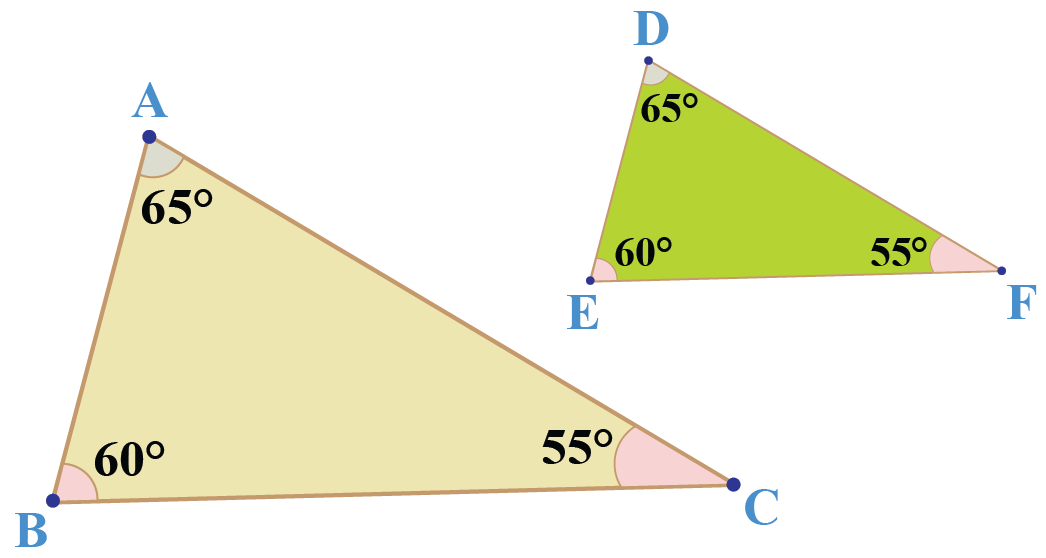Using the AA criterion, we can say that these triangles are similar.

### SSS similarity criterion

The SSS similarity criterion states that if the three sides of one triangle are respectively proportional to the three sides of another, then the two triangles are similar.

This essentially means that any such pair of triangles will be equiangular(All corresponding angle pairs are equal) also.
Consider the following figure, in which the sides of two triangles ($$\Delta ABC$$ and $$\Delta DEF$$) are respectively proportional: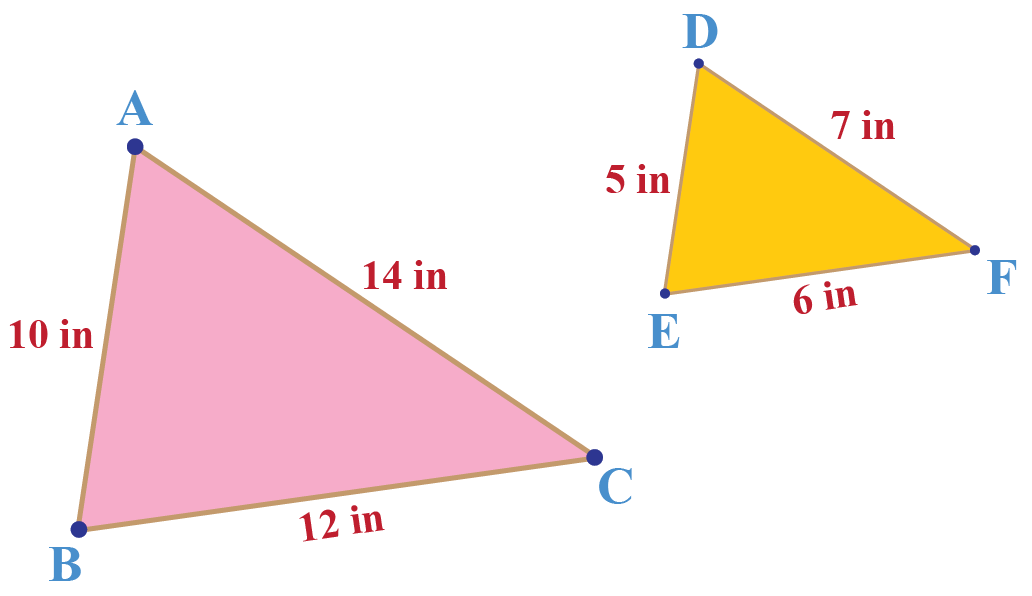That is, it is given that:

$\frac{{AB}}{{DE}} = \frac{{BC}}{{EF}} = \frac{{AC}}{{DF}}$

### SAS similarity criterion

The SAS similarity criterion states that If two sides of one triangle are respectively proportional to two corresponding sides of another, and if the included angles are equal, then the two triangles are similar.

Note the emphasis on the word included. If the equal angle is a non-included angle, then the two triangles may not be similar.
Consider the following figure: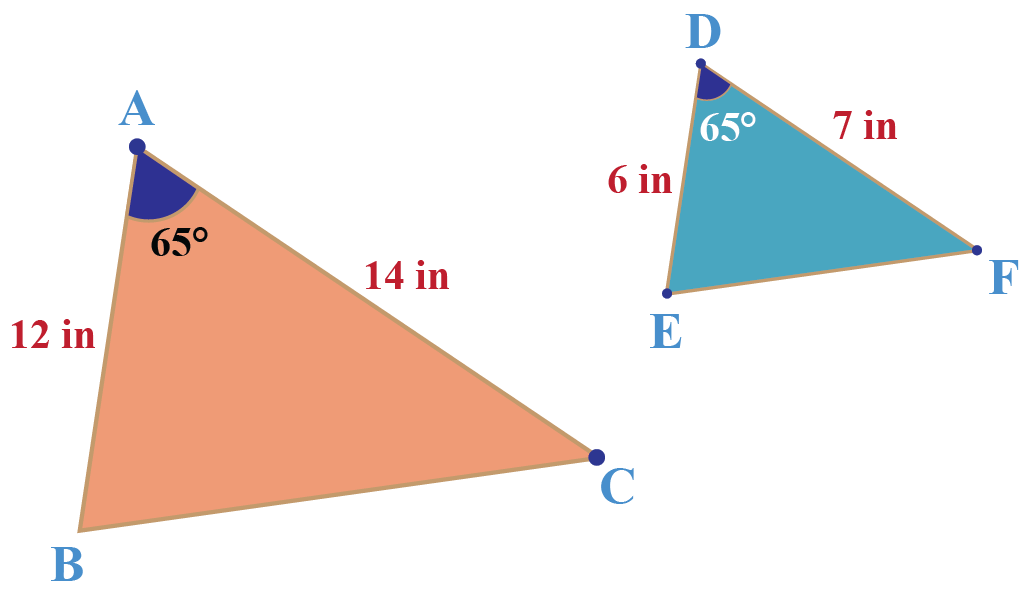It is given that

\begin{align}& \frac{{AB}}{{DE}} = \frac{{AC}}{{DF}} \end{align}

$\angle A = \angle D$

The SAS criterion tells us that $$\Delta ABC$$ ~ $$\Delta DEF$$.

## What Is The Difference Between Similarity And Congruency?

When two figures have the same shape and size, they are congruent. If the figures have the same shape, but not the same size, they are similar.

Look at this pair of coins, they are congruent since having the same shape and the radius of both coins is equal.

Congruent figures are equal in all aspects i.e perimeter, length, and area of congruent figures are always equal.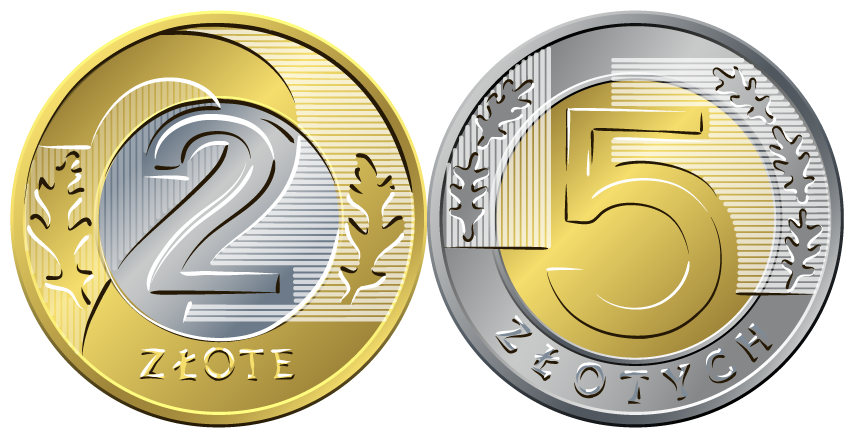The major difference is all congruent figures are similar but similar figures are not congruent.

## What Is The Application Of Similarity?

Some applications of similarity are mentioned below.

• The similarity is widely used in Architecture.
• Solving problems involve height and distance.
• Solving Mathematical problems involving triangles.Important Notes
• If two angles of two triangles are equal then their third angle is always equal.
• The angle bisector of a triangle always divides the triangle into two similar triangles. (Angle Bisector Theorem)
• If two similar triangles have sides in ratio $$\frac{x}{y}$$ then the ratio of their areas will be $$\frac{x^2}{y^2}$$

## Solved Examples

 Example 1

Consider the following figure: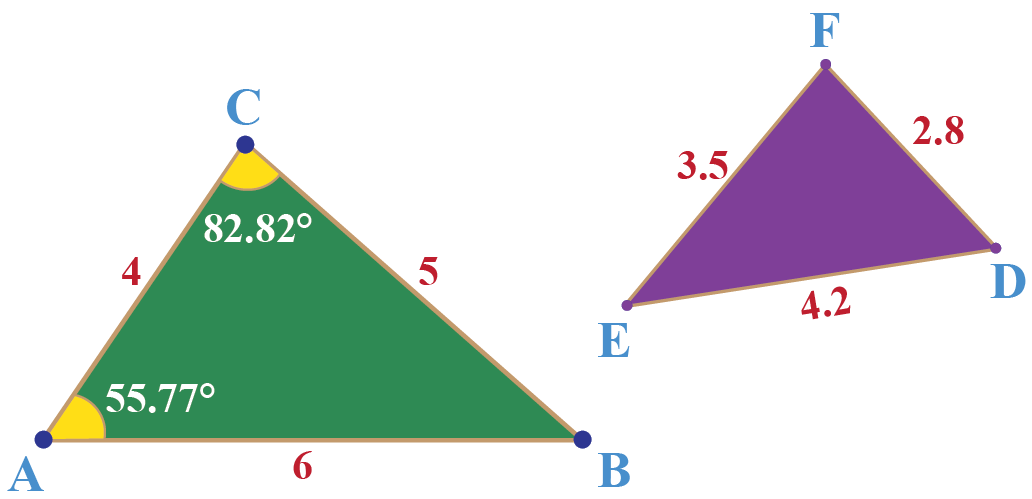Find the value of $$\angle E$$.

Solution

Match the longest side with the longest side and the shortest side with the shortest side and check all three ratios.

We note that the three sides of the two triangles are respectively proportional:

\begin{align}& \left\{ \begin{gathered}\frac{{DE}}{{AB}} = \frac{{4.2}}{6} = 0.7\\ \frac{{DF}}{{AC}} = \frac{{2.8}}{4} = 0.7\\ \frac{{EF}}{{BC}} = \frac{{3.5}}{5} = 0.7 \end{gathered} \right.\\&\quad\frac{{DE}}{{AB}} = \frac{{DF}}{{AC}} = \frac{{EF}}{{BC}} \end{align}

Thus, by SAS similarity criterion, $$\Delta ABC \sim \Delta DEF$$

This means that they are also equi-angular.

Note carefully that the equal angles will be:

$\begin{array}{l} \angle A = \angle D = 55.77^0 \\ \angle C = \angle F = 82.82^0 \\ \angle B = \angle E \end{array}$

Finally,

$\begin{array}{*{20}{l}} {\angle E = \angle B = {{180}^0} - ({{55.77}^0} + {{82.82}^0})} \\ { \Rightarrow \angle E = {{41.41}^0}} \end{array}$

 Example 2

Consider two similar triangles, $$\Delta ABC$$ and $$\Delta DEF$$: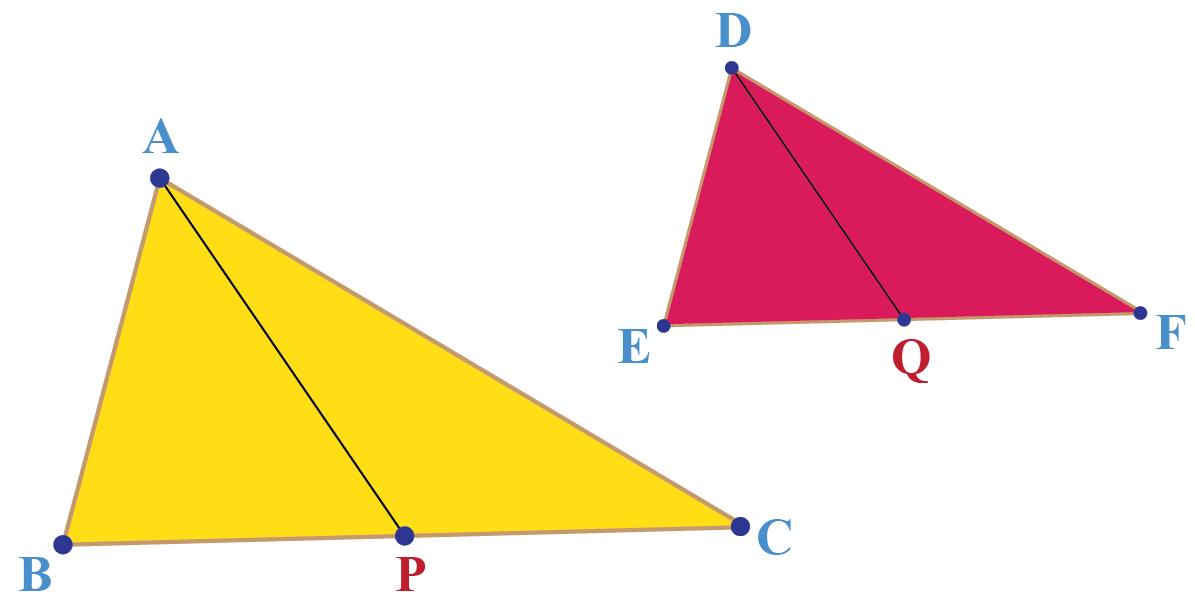$$AP$$ and $$DQ$$ are medians in the two triangles respectively. Show that

$\frac{{AP}}{{BC}} = \frac{{DQ}}{{EF}}$

Solution

Since the two triangles are similar, they are equiangular.

This means that,

$\angle B = \angle E$

Also,

\begin{align} \frac{{AB}}{{DE}} &= \frac{{BC}}{{EF}}\\ \Rightarrow \quad\frac{{AB}}{{DE}} &= \frac{{BC/2}}{{EF/2}} = \frac{{BP}}{{EQ}} \end{align}

Hence, by the SAS similarity criterion,

$\Delta ABP \sim \Delta DEQ$

Thus, the sides of these two triangles will be respectively proportional, and so:

\begin{align} \frac{{AB}}{{DE}} &= \frac{{AP}}{{DQ}}\\ \Rightarrow \quad\frac{{AP}}{{DQ}} &= \frac{{BC}}{{EF}}\\ \Rightarrow \quad\frac{{AP}}{{BC}} &= \frac{{DQ}}{{EF}} \end{align}

 $$\therefore$$ answer statement boxChallenging Questions

Consider the triangle ABC shown in the following figure where RQ = 13 inches, QM = 12  inches, RM = 5 inches cm and $$\angle$$ QRM = $$\angle$$ QPR . What is the ratio of the perimeter of the  $$\Delta$$ PMR to that of the triangle $$\Delta$$ QMR?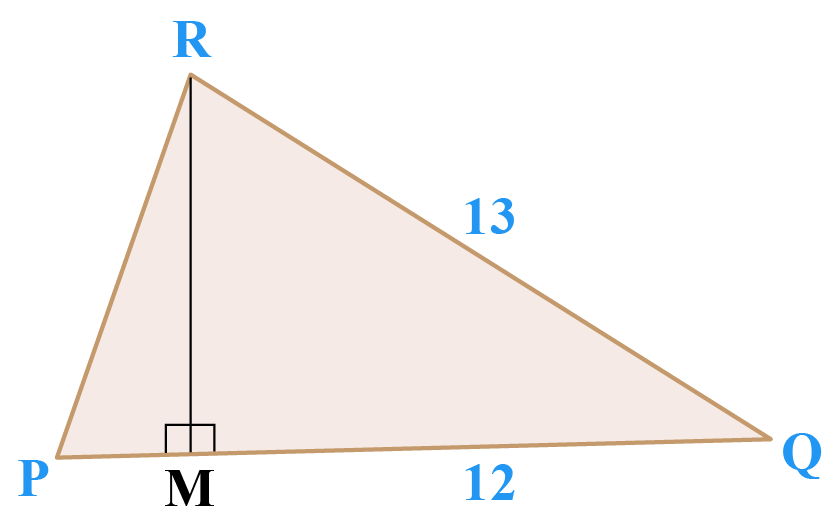## Interactive Questions

Here are a few activities for you to practice. Select/Type your answer and click the "Check Answer" button to see the result.

## Let's Summarize

The mini-lesson targeted the interesting concept of chords of a circle. We hope you enjoyed learning about the diameter and chords of the circle theorem with the simulation and practice questions. Now, equal chords in a circle and unequal chords in a circle are no more difficult for you.

At Cuemath, our team of math experts is dedicated to making learning fun for our favorite readers, the students!
Through an interactive and engaging learning-teaching-learning approach, the teachers explore all angles of a topic.
Be it worksheets, online classes, doubt sessions, or any other form of relation, it’s the logical thinking and smart learning approach that we, at Cuemath, believe in.

## FAQs

### 1.How is similarity used in real life?

The similarity is used in designing, solving the problems involve height and distance, etc.

### 2. What are the rules of similarity?

The three rules of similarity are SSS similarity, SAS similarity, and AA or AAA similarity.

### 3. Is SSA a similarity theorem?

No, SSA is not a similarity theorem.

### 4. What is a similarity statement?

When two or more objects or figures appear the same or equal due to their shape, this property is known as a similarity.

### 5. What is a SSS similarity theorem?

The SSS similarity criterion states that if the three sides of one triangle are respectively proportional to the three sides of another, then the two triangles are similar.

### 6. What is a SAS similarity theorem?

The SAS similarity criterion states that If two sides of one triangle are respectively proportional to two corresponding sides of another, and if the included angles are equal, then the two triangles are similar.

### 7. What is a AA similarity theorem?

The AA criterion for triangle similarity states that if the three angles of one triangle are respectively equal to the three angles of the other, then the two triangles will be similar.

Similar Triangles
Similar Triangles
grade 9 | Questions Set 2
Similar Triangles
Similar Triangles
grade 9 | Questions Set 1
More Important Topics
Numbers
Algebra
Geometry
Measurement
Money
Data
Trigonometry
Calculus
More Important Topics
Numbers
Algebra
Geometry
Measurement
Money
Data
Trigonometry
Calculus